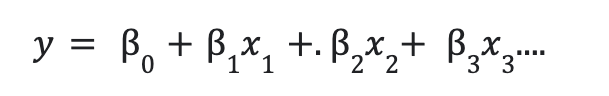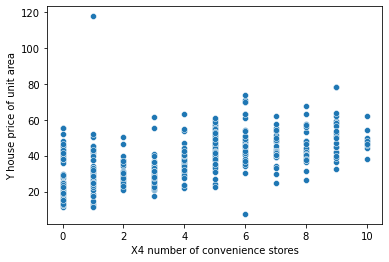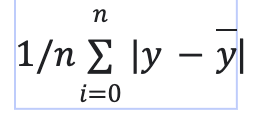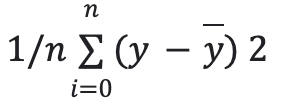# Multiple Linear Regression With scikit-learn

• Last Updated : 11 Jul, 2022

In this article, let’s learn about multiple linear regression using scikit-learn in the Python programming language.

Regression is a statistical method for determining the relationship between features and an outcome variable or result. Machine learning, it’s utilized as a method for predictive modeling, in which an algorithm is employed to forecast continuous outcomes. Multiple linear regression, often known as multiple regression, is a statistical method that predicts the result of a response variable by combining numerous explanatory variables. Multiple regression is a variant of linear regression (ordinary least squares)  in which just one explanatory variable is used.

Mathematical Imputation:

To improve prediction, more independent factors are combined. The following is the linear relationship between the dependent and independent variables:here, y is the dependent variable.

• x1, x2,x3,… are independent variables.
• b0 =intercept of the line.
• b1, b2, … are coefficients.

for a simple linear regression line is of the form :

y = mx+c

for example if we take a simple example, :

feature 1: TV

feature 3:  Newspaper

output variable: sales

Independent variables are the features feature1 , feature 2 and feature 3. Dependent variable is sales. The equation for this problem will be:

y = b0+b1x1+b2x2+b3x3

x1, x2 and x3 are the feature variables.

In this example, we use scikit-learn to perform linear regression. As we have multiple feature variables and a single outcome variable, it’s a Multiple linear regression. Let’s see how to do this step-wise.

## Stepwise Implementation

### Step 1: Import the necessary packages

The necessary packages such as pandas, NumPy, sklearn, etc… are imported.

## Python3

 `# importing modules and packages``import` `pandas as pd``import` `numpy as np``import` `matplotlib.pyplot as plt``import` `seaborn as sns``from` `sklearn.model_selection ``import` `train_test_split``from` `sklearn.linear_model ``import` `LinearRegression``from` `sklearn.metrics ``import` `mean_squared_error, mean_absolute_error``from` `sklearn ``import` `preprocessing`

### Step 2: Import the CSV file:

The CSV file is imported using pd.read_csv() method. To access the CSV file click here. The ‘No ‘ column is dropped as an index is already present. df.head() method is used to retrieve the first five rows of the dataframe. df.columns attribute returns the name of the columns. The column names starting with ‘X’ are the independent features in our dataset. The column ‘Y house price of unit area’ is the dependent variable column. As the number of independent or exploratory variables is more than one, it is a Multilinear regression.

## Python3

 `# importing data``df ``=` `pd.read_csv(``'Real estate.csv'``)``df.drop(``'No'``, inplace ``=` `True``,axis``=``1``)`` ` `print``(df.head())``print``(df.columns)`

Output:

X1 transaction date  X2 house age  …  X6 longitude  Y house price of unit area

0             2012.917          32.0  …     121.54024                        37.9

1             2012.917          19.5  …     121.53951                        42.2

2             2013.583          13.3  …     121.54391                        47.3

3             2013.500          13.3  …     121.54391                        54.8

4             2012.833           5.0  …     121.54245                        43.1

[5 rows x 7 columns]

Index([‘X1 transaction date’, ‘X2 house age’,

‘X3 distance to the nearest MRT station’,

‘X4 number of convenience stores’, ‘X5 latitude’, ‘X6 longitude’,

‘Y house price of unit area’],

dtype=’object’)

### Step 3: Create a scatterplot to visualize the data:

A scatterplot is created to visualize the relation between the ‘X4 number of convenience stores’ independent variable and the ‘Y house price of unit area’ dependent feature.

## Python3

 `# plotting a scatterplot``sns.scatterplot(x``=``'X4 number of convenience stores'``,``                ``y``=``'Y house price of unit area'``, data``=``df)`

Output:### Step 4: Create feature variables:

To model the data we need to create feature variables, X variable contains independent variables and y variable contains a dependent variable. X and Y feature variables are printed to see the data.

## Python3

 `# creating feature variables``X ``=` `df.drop(``'Y house price of unit area'``,axis``=` `1``)``y ``=` `df[``'Y house price of unit area'``]``print``(X)``print``(y)`

Output:

X1 transaction date  X2 house age  …  X5 latitude  X6 longitude

0               2012.917          32.0  …     24.98298     121.54024

1               2012.917          19.5  …     24.98034     121.53951

2               2013.583          13.3  …     24.98746     121.54391

3               2013.500          13.3  …     24.98746     121.54391

4               2012.833           5.0  …     24.97937     121.54245

..                   …           …  …          …           …

409             2013.000          13.7  …     24.94155     121.50381

410             2012.667           5.6  …     24.97433     121.54310

411             2013.250          18.8  …     24.97923     121.53986

412             2013.000           8.1  …     24.96674     121.54067

413             2013.500           6.5  …     24.97433     121.54310

[414 rows x 6 columns]

0      37.9

1      42.2

2      47.3

3      54.8

4      43.1

…

409    15.4

410    50.0

411    40.6

412    52.5

413    63.9

Name: Y house price of unit area, Length: 414, dtype: float64

### Step 5: Split data into train and test sets:

Here, train_test_split() method is used to create train and test sets, the feature variables are passed in the method. test size is given as 0.3, which means 30% of the data goes into test sets, and train set data contains 70% data. the random state is given for data reproducibility.

## Python3

 `# creating train and test sets``X_train, X_test, y_train, y_test ``=` `train_test_split(``    ``X, y, test_size``=``0.3``, random_state``=``101``)`

### Step 6: Create a linear regression model

A simple linear regression model is created. LinearRegression() class is used to create a simple regression model, the class is imported from sklearn.linear_model package.

## Python3

 `# creating a regression model``model ``=` `LinearRegression()`

### Step 7: Fit the model with training data.

After creating the model, it fits with the training data. The model gains knowledge about the statistics of the training model. fit() method is used to fit the data.

## Python3

 `# fitting the model``model.fit(X_train,y_train)`

### Step 8: Make predictions on the test data set.

In this model.predict() method is used to make predictions on the X_test data, as test data is unseen data and the model has no knowledge about the statistics of the test set.

## Python3

 `# making predictions``predictions ``=` `model.predict(X_test)`

### Step 9: Evaluate the model with metrics.

The multi-linear regression model is evaluated with mean_squared_error and mean_absolute_error metric. when compared with the mean of the target variable, we’ll understand how well our model is predicting. mean_squared_error is the mean of the sum of residuals. mean_absolute_error is the mean of the absolute errors of the model. The less the error, the better the model performance is.

mean absolute error = it’s the mean of the sum of the absolute values of residuals.mean square error =  it’s the mean of the sum of the squares of residuals.• y= actual value
• y hat = predictions

## Python3

 `# model evaluation``print``(``  ``'mean_squared_error : '``, mean_squared_error(y_test, predictions))``print``(``  ``'mean_absolute_error : '``, mean_absolute_error(y_test, predictions))`

Output:

```mean_squared_error :  46.21179783493418
mean_absolute_error :  5.392293684756571```

For data collection, there should be a significant discrepancy between the numbers. If you want to ignore outliers in your data, MAE is a preferable alternative, but if you want to account for them in your loss function, MSE/RMSE is the way to go. MSE is always higher than MAE in most cases, MSE equals MAE only when the magnitudes of the errors are the same.

### Code:

Here, is the full code together, combining the above steps.

## Python3

 `# importing modules and packages``import` `pandas as pd``import` `numpy as np``import` `matplotlib.pyplot as plt``import` `seaborn as sns``from` `sklearn.model_selection ``import` `train_test_split``from` `sklearn.linear_model ``import` `LinearRegression``from` `sklearn.metrics ``import` `mean_squared_error, mean_absolute_error``from` `sklearn ``import` `preprocessing`` ` `# importing data``df ``=` `pd.read_csv(``'Real estate.csv'``)``df.drop(``'No'``, inplace``=``True``, axis``=``1``)`` ` `print``(df.head())`` ` `print``(df.columns)`` ` `# plotting a scatterplot``sns.scatterplot(x``=``'X4 number of convenience stores'``,``                ``y``=``'Y house price of unit area'``, data``=``df)`` ` `# creating feature variables``X ``=` `df.drop(``'Y house price of unit area'``, axis``=``1``)``y ``=` `df[``'Y house price of unit area'``]`` ` `print``(X)``print``(y)`` ` `# creating train and test sets``X_train, X_test, y_train, y_test ``=` `train_test_split(``    ``X, y, test_size``=``0.3``, random_state``=``101``)`` ` `# creating a regression model``model ``=` `LinearRegression()`` ` `# fitting the model``model.fit(X_train, y_train)`` ` `# making predictions``predictions ``=` `model.predict(X_test)`` ` `# model evaluation``print``(``'mean_squared_error : '``, mean_squared_error(y_test, predictions))``print``(``'mean_absolute_error : '``, mean_absolute_error(y_test, predictions))`

Output:

X1 transaction date  X2 house age  …  X6 longitude  Y house price of unit area

0             2012.917          32.0  …     121.54024                        37.9

1             2012.917          19.5  …     121.53951                        42.2

2             2013.583          13.3  …     121.54391                        47.3

3             2013.500          13.3  …     121.54391                        54.8

4             2012.833           5.0  …     121.54245                        43.1

[5 rows x 7 columns]

Index([‘X1 transaction date’, ‘X2 house age’,

‘X3 distance to the nearest MRT station’,

‘X4 number of convenience stores’, ‘X5 latitude’, ‘X6 longitude’,

‘Y house price of unit area’],

dtype=’object’)

X1 transaction date  X2 house age  …  X5 latitude  X6 longitude

0               2012.917          32.0  …     24.98298     121.54024

1               2012.917          19.5  …     24.98034     121.53951

2               2013.583          13.3  …     24.98746     121.54391

3               2013.500          13.3  …     24.98746     121.54391

4               2012.833           5.0  …     24.97937     121.54245

..                   …           …  …          …           …

409             2013.000          13.7  …     24.94155     121.50381

410             2012.667           5.6  …     24.97433     121.54310

411             2013.250          18.8  …     24.97923     121.53986

412             2013.000           8.1  …     24.96674     121.54067

413             2013.500           6.5  …     24.97433     121.54310

[414 rows x 6 columns]

0      37.9

1      42.2

2      47.3

3      54.8

4      43.1

…

409    15.4

410    50.0

411    40.6

412    52.5

413    63.9

Name: Y house price of unit area, Length: 414, dtype: float64

mean_squared_error :  46.21179783493418

mean_absolute_error :  5.392293684756571

My Personal Notes arrow_drop_up